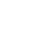Shaders and Functions

The Shading Language distinguishes between shaders and functions. Shaders are procedures that are referred to by RenderMan Interface procedures. Functions are procedures that can be called from within the Shading Language. The distinction between shaders and functions is primarily a consequence of different argument passing conventions.

Shaders are introduced by the keywords light, surface, volume, displacement, or imager and are followed by the name of the shader and the statements that comprise its definition. These keywords indicate the type of RenderMan Interface shader that is being defined. The RenderMan Interface uses the shader type to enforce a type match in subsequent calls to RenderMan Interface procedures. For example, it is illegal to declare a shader to be of type light and then instance it using RiSurface.

The arguments to a shader are referred to as its instance variables. All of these variables are required have default values, and are assumed to be uniform unless declared otherwise. Shader instance variables can be changed when a particular shader is instanced from the RenderMan Interface. For example, consider the shader weird:

surface
weird( float a=0.5; varying float b=0.25 )
{
Ci = color (mod(s,a),  abs(sin(a+b)),  mod(b,t));
}

This surface shader may be referenced through the RenderMan Interface with the RiSurface command. For example,

RiSurface( "weird", RI_NULL );

instances the above shader with all its defaults.

Shader instance variable values can be changed from their defaults by passing their new values through the RenderMan Interface. This first requires that the type of the variable be declared. The declaration for weird would be:

RiDeclare( "a", "uniform float" );
RiDeclare( "b", "varying float" );

Of course, the RiDeclare can be eliminated if "in-line declaraions" are used (see Section 3).  In either case, uniform instance variables can be set by passing them in the parameterlist of a shader instance.  In the following example a is redefined while b remains equal to its default.

RtFloat a = 0.3;
RiSurface( "weird", "a", (RtPointer)&a, RI_NULL );

Shader variables can also be set in geometric primitives. For example, the weird shader variables could be set when defining a primitive:

RtFloat	a;
RtFloat	bs;
RtPoint	Ps;
RiPolygon(4, "P", Ps, "a", (RtPointer)&a, "b", (RtPointer)bs, RI_NULL)

a is a single float and b is an array containing four values, since it is a varying variable. The standard variable "P" is predeclared to be of type varying point.

If a geometric primitive sets a shader variable that is defined in none of the shaders associated with that primitive, it is ignored. Variables that are set on geometric primitives override the values set by the shader instance.

Shader instance variables are read-only in the blody of the shader, unless they are declared using the output keyword:

displacement
lumpy( float a=0.5; output varying float height=0 )
{
float h = a * noise(P);
P += h * normalize(N);
N = calculatenormal(N);
height = h;
}

This displacement shader produces a lumpy surface and also saves the displacement amount in an output variable height.  Output variables of one shader may be read by other shaders on the same primitive (see Section 15.8) in order to pass information between them.

Functions

Functions are similar to shaders except they can be called from shaders or other function but cannot be instanced from the RenderMan Interface. Functions in the Shading Language are also much like functions in C. For example, the following defines a function normalize that returns a unit vector in the direction V:

point
normalize ( vector V )
{
return V/length(V);
}

Function calls use the traditional C syntax, except for the issues noted below.

Functions may return any of the basic types (float, color, point, vector, normal, matrix, string).  Functions may not return arrays, though they may take arrays as parameters.  Functions must have exactly one return statement, except for those functions declared with type void, which may note have any return statement.

Function parameters are passed by reference; in other words, parameters are not copied into private areas of the function.  Nevertheless, function parameters are not writable unless specified with the output keyword.  For example:

void
normalize_inplace ( output vector V )
{
V = V/length(V);
}

Functions in the Shading Language cannot be called recursively.

Functions may be polymorphic - that is, you may define multiple functions with the same name, as long as they take different types or numbers or arguments.  Functions may even be polymorphic based on return type.  For example:

float
snoise ( point p )
{
return 2 * (float noise(p)) - 1;
}
float snoise( float s, float t )
{
return 2 * (float noise(s,t)) - 1;
}
vector snoise(point p)
{
return 2 * (vector noise(p)) - 1;
}

Functions may be declared anyplace that it is legal to declare a variable or have any other language statement, including inside the body of a shaders, inside another function, or inside any other code block.  A function may only be called within the lexical scope in which it is declared, and only after its declaration (the same rules that apply to using a declared variables).  The only data that functions may access are those passed as parameters, except for variables declared with the extern keyword, which indicates that the variable is in an outer scope rather than that local storage should be allocated.  For example:

surface mysurf ( float Knoise = 0.5; ) /* parameter */
{
{
extern float Knoise;
return Knoise * color noise(transform("shader", p));
}
/* code for the shader */